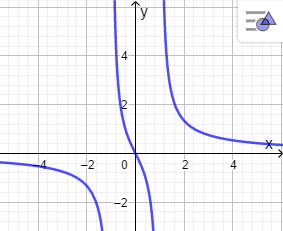# Consider f(x) = \frac{2x}{x^2 - 1}. (A) Where are the zeros of the function? (B) On what...

## Question:

Consider {eq}f(x) = \frac{2x}{x^2 - 1}. {/eq}

(A) Where are the zeros of the function?

(B) On what intervals is this function increasing?

(C) Where are the local maxima and minima?

(D) it is a fact that {eq}f''(x) = 4x \frac{x^2 + 3}{(x^2 - 1)^3} {/eq}. On what intervals is the function concave up?

(E) Where are the inflection points?

(F) Find all vertical and horizontal asymptotes if there is any.

(G) Using the information above, sketch a graph of the function.

## Concavity and inflection points

The graph of the function allows us to observe the behavior, if the function increases or decreases, with the second derivative of the function we can calculate the inflection points that the function may have.

We have the function

{eq}f(x) = \frac{2x}{x^2 - 1} \\ {/eq}

(A) Where are the zeros of the function?

We equalize the function to zero.

{eq}\frac{2x}{x^2 - 1} =0 \\ x=0 \\ {/eq}

(B) On what intervals is this function increasing?

Differentiating the function

{eq}f'(x)=-2\,{\frac {{x}^{2}+1}{ \left( {x}^{2}-1 \right) ^{2}}} \\ {/eq}

{eq}f'(x)=0 {/eq} The function has no real solution. The function has no critical points.

Therefore,

{eq}\begin{array}{r|D{.}{,}{5}} Interval & {-\infty<x<-1} & {-1<x<1} & {1<x<\infty} \\ \hline Test \space{} value & \ x=-2 & \ x=0 & \ x=2 \\ Sign \space{} of \ f'(x) & \ f'(-2)<0 & \ f'(0)<0 & \ f'(2)<0 \\ Conclusion & decreasing & decreasing & decreasing \\ \end{array} \\ {/eq}

The function is not increasing.

(C) Where are the local maxima and minima?

The function has no critical points, therefore, it has no relative extremes.

(D) it is a fact that {eq}f''(x) = 4x \frac{x^2 + 3}{(x^2 - 1)^3} {/eq}. On what intervals is the function concave up?

Differentiating the function

{eq}f'(x)=-2\,{\frac {{x}^{2}+1}{ \left( {x}^{2}-1 \right) ^{2}}} \\ f''(x)=4x \frac{x^2 + 3}{(x^2 - 1)^3} \\ {/eq}

{eq}f''(x)=0 {/eq} when {eq}x=0 {/eq}

{eq}\begin{array}{r|D{.}{,}{5}} Interval & {-\infty<x<0} & {0<x<\infty} \\ \hline Test \space{} value & \ x=-2 & \ x=2 \\ Sign \space{} of \ f'' (x) & \ f'' (-2)<0 & \ f'' (2)>0 \\ Conclusion & concave \space down & concave \space up \\ \end{array} \\ {/eq}

(E) Where are the inflection points?

{eq}(0,0) \\ {/eq}

(F) Find all vertical and horizontal asymptotes if there is any.

Vertical asymptotes:

{eq}x^{2}-1=0 {/eq}

{eq}\begin{array}{l} \begin{array}{l} \text{This is a}\space{}{\text{second degree}}\space{}\text{equation with the form:}\space{}a x ^2+b x + c=0,\space{}\text{where}\space{}a= 1 ,\space{}b= 0 \space{}\text{and}\space{}c= -1 .\space{}\text{The solution is:} \end{array} \\ \begin{array}{lcl} x &=&\frac{-b\pm\sqrt{b^2-4*a*c}}{2*a} \\ &=&\frac{ - ( 0 ) \pm\sqrt{ ( 0 )^2 - 4 ( 1 ) ( -1 )}}{ 2 ( 1 ) } \\ &=&\frac{ 0 \pm\sqrt{ 0 - ( -4 ) }} { 2 } \\ &=&\frac{ 0 \pm\sqrt{ 0 + 4 }} { 2 } \\ &=&\frac{ 0 \pm\sqrt{ 4 }} { 2 } \\ &=&\frac{ 0 \pm 2 } { 2 } \end{array} \\ \text{First solution:} \\ \begin{array}{lcl} x _1&=&\frac{ 0 + 2 } { 2 } \\ &=&\frac{ 2 } { 2 } \\ &=& 1 \end{array} \\ \text{Second solution:} \\ \begin{array}{lcl} x _2&=&\frac{ 0 - 2 } { 2 } \\ &=&\frac{ -2 } { 2 } \\ &=& -1 \end{array} \end{array} {/eq}

Horizontal asymptotes:

{eq}\displaystyle{\lim_{ x \rightarrow \infty}} f(x) \\ \displaystyle{\lim_{ x \rightarrow \infty}} \frac{2x}{x^2 - 1} \\ y=0 \\ {/eq}

(G) Using the information above, sketch a graph of the function.

Graph the function